# In situ single-atom array synthesis using dynamic holographic optical tweezers

## Abstract

Establishing a reliable method to form scalable neutral-atom platforms is an essential cornerstone for quantum computation, quantum simulation and quantum many-body physics. Here we demonstrate a real-time transport of single atoms using holographic microtraps controlled by a liquid-crystal spatial light modulator. For this, an analytical design approach to flicker-free microtrap movement is devised and cold rubidium atoms are simultaneously rearranged with 2N motional degrees of freedom, representing unprecedented space controllability. We also accomplish an in situ feedback control for single-atom rearrangements with the high success rate of 99% for up to 10 μm translation. We hope this proof-of-principle demonstration of high-fidelity atom-array preparations will be useful for deterministic loading of N single atoms, especially on arbitrary lattice locations, and also for real-time qubit shuttling in high-dimensional quantum computing architectures.

## Introduction

In this regard, using holographic optical tweezers is advantageous because arbitrary potentials can be designed for atoms6,12. The optical tweezers are the image determined by the wave propagation integral, for example, Fourier transformation, so the hologram for a complex potential can be designed using a numerical method often based on iterative Fourier transformation algorithms (IFTAs)12. When being used in conjunction with an active holographic device such as spatial light modulators (SLM), the algorithms can produce dynamic optical potentials, of which the many applications include dynamic in situ atom manipulation20,21,22,23, quantum logic gate24, pattern formations in an addressable optical lattice19 and real-time feedback transportation of atoms7. However, there has been no scheme implemented for the rearrangement of many atoms, with the full degree of control (2N for N atoms) in two-dimensional (2D) space.

In this paper, we demonstrate a dynamic holographic single-atom tweezer with unprecedented degrees of freedom of 2N. In a proof-of-principle experiment conducted with cold 87Rb atoms, simultaneous rearrangements of up to N=9 single atoms were successfully performed. This method may be further applicable to deterministic N single-atom loading, coherent transport20,21 and controlled collisions22,23.

## Results

### Experimental concept and flicker-free beam steering algorithm

Figure 1 shows the concept of our experiment and the first demonstration of N single-atom transport with 2N motional degrees of freedom. The setup consists of an active holographic device (liquid-crystal SLM (LC-SLM)), an imaging system and a cold 87Rb atom chamber, as shown in Fig. 1a. When the holograms were transferred by a two-lens system to the entrance pupil of a high numerical aperture (NA=0.5) lens12, the optical tweezers had a beam radius of wo=1.14 μm and the trap frequencies were measured as fr=100 kHz and fz=17 kHz for radial and axial directions, respectively25. The temperature of the trapped atoms was measured as T=110(10) μK using the release and recapture method26, where the error was the 1σ band of each optical tweezer.

Schematic examples of our trap generation algorithm are represented in Fig. 1b. Our algorithm uses the simplest analytic form of beam steering, that is, the linear phase ϕ(x)=kxx. This phase modulation directly couples the modulation plane (Fourier domain) control parameter, kx, to the image plane position, X=Fkx/k, with one-to-one correspondence, where F is the lens focal length and k is the wave-vector of the trap light. Then, the given function becomes a flicker-free solution, because a linear combination of two phases, αk1x+(1−α)k2x with α[0, 1], is again a linear phase which smoothly sweeps the two focal points, X1=Fk1/k and X2=Fk2/k (see Methods for more details). To obtain more than a single optical tweezer, the modulation plane is divided into several sub-planes as in Fig. 1b27. When each sub-plane is assigned to each linear phase and the division is randomized in the single-pixel resolution of the device, this method effectively preserves the diffraction limit of the individual optical tweezers focused onto the image plane. Note that the required trap laser power in this manner scales with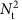, where Nt is the number of optical tweezers; hence, it is inefficient compared with the IFTA, which scales with Nt because the IFTA coherently sums over the entire modulation plane for every tweezer. Nevertheless, the proposed algorithm concedes power efficiency for independent controllability.

### Simultaneous transport of N single atoms

An image accumulating 500 snapshots from different loading events is shown in Fig. 1c. The three-dimensional (3D) molasses continuous imaging10,28 captured a snapshot every 60 ms with 50 ms exposure time, where the single-event atom visibility exceeded 99.99%. If Nt increase, the number of SLM pixels per single optical tweezer would decrease, thus degrading the optical tweezer shape and intensity regularity. In Fig. 1d, the deviation of the normalized peak intensity δ (the y axis) is given proportional to the number of tweezers Nt (the x axis) and inversely proportional to the beam waist W, that is, δ(Nt−1)/W. This behaviour can be understood as following: the peak intensity of each tweezer follows the binomial distribution B(M, 1/Nt), where 1/Nt is the success probability and MW2 is the number of active SLM pixels, so the normalized variance is given by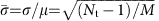, where the mean and variance are μ=M/Nt and σ2=M(Nt−1)/, respectively. In addition, there are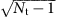events that equally contribute to this normalized variance, so we get δ(Nt−1)/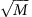(Nt−1)/W. To maintain an acceptable quality of the optical tweezers for the experiments, we chose N=9 and W=2 mm (the beam waist), limited by the laser power and relay optics dimensions, respectively. In our experiment, the standard deviation of the normalized peak intensity below 0.02 is tested, and a setup with a bigger laser power would support more optical tweezers. Note that, because of thepower-scaling, diffused light will eventually wash out the tweezer potential and the maximally available tweezers in our method is estimated to be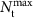=100. Figure 1e shows an array-rearrangement demonstration. The series of images each accumulating also 500 snapshots of the individual experiments demonstrates that the initially prepared a 3-by-3 square array of single atoms with a spacing of d=4.5 μm expands to an array of twice the lattice spacing of 2d. Figure 2 shows a single-atom transport example; in this case, N single atoms are moved from an initial array along a predefined path. Because each atom moves in 2D with parameters (Xi, Yi) for i=1 to N=9, the total degrees of freedom of movement is 2N=18.

### Single-atom array synthesis demonstration

A proof-of-principle demonstration of the in situ single-atom array synthesis is presented in Fig. 3. In a three-step feedback loop of initial atom loading in Ninit=9 sites, readout, and rearrangement, as shown in Fig. 3a, an atom array of Nfinal=1, 2, 3 or 4, is produced. After the atoms are initially loaded at the sites, the first computer checks the occupancy of each site by reading out the electron multiplying charge-coupled device (CCD) images. A 9-bit binary information that represents the occupancy is then sent to the second computer, which has a look-up table of atom rearrangement trajectories, each stored in DRAM as a sequence of 30 holograms, between all possible initial and final pairs of atom arrays. It takes 0.6 s from the readout to the retrieval of an appropriate trajectory (note that, using a fast graphic-processing unit can greatly reduce this time and also make the look-up table unnecessary29). Then, the second computer sequentially loads the holograms to the SLM at a speed of 30 frame-per-second to move the atoms along the trajectory. The initial, and four types of final cumulative images are represented by the atom number histograms in Fig. 3b,c. The individual final images are independent experiments that form one, two, three or four atom arrays out of nine.

Compared with the binomial distribution of the initial histogram, the final histogram in Fig. 3d has non-Poissonian distribution. The loading efficiency curves of the final arrays are presented with red points for the data from the number histograms of at least 500 events. The black dotted line follows 0.5N, which is the loading efficiency in the collisional blockade regime, and the blue dashed line follows the cumulative binomial distribution given by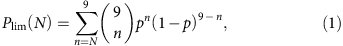and the red line iswhere p=0.48 is the initial loading probability and ps=0.86 is the experimental (moving) success probability from the fitting. 1−ps is composed of the background collisional and moving losses. During the entire feedback process, the background collisional loss is estimated to be 0.13 and the moving loss is estimated to be 0.01. The moving success rate, as expected, has high fidelity and a deterministic transport has been reliably completed. The Nfinal=4 case exhibits sixfold enhancement in loading efficiency, compared with the P=0.5 collisional blockade regime.

## Discussion

The physics behind this demonstration is the capability of holographic optical tweezers to sustain trapped atoms while the hologram is being actively updated, but this has been considered impossible because of intensity flickering30,31. If conventional IFTAs are used to generate individual holograms to form the required optical potentials, the frame-to-frame evolution does not necessarily maintain an appropriate in-between potential (see Fig. 4a). While the intensity flickering is not an issue for macroscopic particles suspended in a solution32, microscopic particles (for example, atoms) do not wait until the missing potential recovers or cannot resist excessive displacement heating. Even with a fast device such as a digital micromirror device (50 kHz frame)33 or for ultracold atoms34, a large portion of the trapped atoms is lost. The trap loss simulation (see Methods) performed as a function of the frame rate, f, of the device and the trap frequency,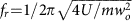, supports the idea that the intensity flickering hinders the trap stability (see Fig. 4b,c). In particular, a constant loss exists because of the significant intensity flickering in the adiabatic region (frf, Region 1 in Fig. 4c). In the non-adiabatic region (fr<f, Region 2 in Fig. 4c), single steps do not lose atoms (because the intensity flickering is fast enough); however, in this region, either the atoms boil up fast via displacement heating or current technologies are not applicable. The holographic transport of single atoms, therefore, requires an alternative algorithmic approach (this work) to reduce the intensity flickering.

Our method can be further improved by increasing the number of atoms and the efficiency of initial loading. Besides simply increasing the number of dynamic holographic tweezers, one may use a passive diffractive optical element (DOE) in addition. A commonly available 6-by-6 passive DOE, for example, can capture N=9 atoms with a probability exceeding 99.8% at P=0.48, so subsequently an active element (SLM) can pick up and move them by taking the full advantage of ‘all’ available SLM tweezers. Also, the more stable traps, such as passive DOE or acousto-optic modulators, can be used to store them for an extended time35,36. (Note that the acousto-optic modulator-based method for 2D transportation uses N1+N2 diffracted beams to make an array of N1 × N2 tweezers, so the moving degrees of freedom in that case is limited by N1+N2.) Furthermore, lowering the background collision can further improve the performance: in a low-pressure (10−11 Torr) chamber that allows ps=0.97, the probability of creating a completely packed 3-by-3 atom array is expected to be as high as 80%.

In summary, the analytic approach to optical potential design has demonstrated the holographic transport of single-atom arrays. The systematic analysis of intensity flicker enabled the moving loss to be parameterized; thus, we could find and achieve the deterministic transport regime using a holographic method. An individual atom has its own degrees of freedom in the image plane; thus, total moving degrees of freedom of 2N was achieved, which demonstrates unprecedented space controllability. Furthermore, overlapping Fresnel lens pattern37 can transport the array in the axial direction, suggesting that total 3N degrees of freedom is also possible. We also formed an in situ feedback loop for atom array rearrangement, which is a proof-of-principle demonstration of high-fidelity atom array preparation. The possible application is not limited to the deterministic array preparation but may be extended to many-body physics with arranged atoms and coherent qubit transports.

## Methods

### Experiments

The active holographic device was a liquid-crystal SLM (HOLOEYE, PLUTO), a reflective phase modulator array of 1,920 × 1,080 pixels with an 8 μm2 pixel size and the first-order diffraction efficiency was 50%. The far-off-resonant trap (FORT) beam of Ptotal=1.1 W from a Ti:sapphire continuous-wave laser (M Squared, SolsTiS) was tuned at λ=820 nm to illuminate the SLM with a beam radius of W=2 mm in a near-orthogonal incident angle. The diffracted beam from the SLM was imaged onto the intermediate image plane by an F1=200 mm lens, and then re-imaged onto the entrance pupil of the objective lens by a second lens (doublet, F2=200 mm). The objective lens (Mitutoyo, G Plan Apo) was an infinity-corrected system, having a focal length of F3=4 mm, a numerical aperture of NA=0.5, and a long working distance of 16 mm with 3.5 mm-thick glass-plate compensation. Then the given laser power was able to sustain up to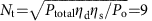optical tweezers with a trap depth of U=1.4 mK, where the diffraction efficiency was ηd=0.5, the optical system loss ηs=0.5, and the optical power Po=3.4 mW.

The cold 87Rb atom chamber was a dilute vapour glass cell in a constant pressure of 3 × 10−10 Torr. It had four 100 × 40 mm2 clear windows with a thickness of 3.5 mm. The 87Rb atoms from a getter were captured by a six-arm 3D magneto-optical trap with a beam diameter of 7.5 mm (1/e2), a detuning of −18 MHz from the 5S1/2(F=2)→5P3/2(F ′=3) hyperfine transition, and dB/dz=15 G cm−1. After an initial magneto-optical trap loading operation for 2.8 s, the atom density became 1010 cm−3 (equivalent to 0.2 atoms per single trap volume), so the −46-MHz detuned 3D molasses and the FORT were overlapped for 200 ms to achieve the collisional blockade regime of the P=0.5 filling probability in every site10. After this, the magnetic-field gradient and the molasses were turned off for 100 ms to dissipate residual cold atoms and then the 3D molasses were turned back on for continuous imaging. The scattered photons were collected by the same objective lens F3 and imaged onto the CCD (Andor, iXon3 897) through the lens F2 with an overall efficiency of ηc=0.02. The image plane of 26 × 26 μm2 was captured as a snapshot in every 60 ms. The trap lifetime was measured as 12 s, consistent with the effect of the background gas collision.

### Single-atom detection

The scattering cross-section is given by σ=σ0/[1+4(Δ/Γ)2+I/Isat], where the resonant cross-section is σ0=2.907 × 10−9 cm2, the natural line width Γ=5.746 MHz, the saturation intensity Isat=1.669 mW cm−2, the detuning Δ=−100 MHz (Stark shift is considered), and the intensity I=27 mW cm−2. Each atom in an optical tweezer emits 2.87 × 105 photons per second. With the overall detection efficiency of 0.02 and the exposure time of 50 ms, we collect 280 photons per atom. It corresponds to a signal-to-noise ratio >5 for the CCD. When a Gaussian noise is assumed, the theoretical discrimination probability between zero and single atom is given with 5σ significance or 99.99995%. In the experiments, a background photon noise exists, but the histogram shows the success probability exceeding 99.99%.

### Heating in optical tweezers

There are several heating sources for trapped atoms including the FORT scattering, the intensity noise, the beam pointing fluctuation; however, none of them are a significant heating source. The heating caused by the photon scattering from FORT is estimated as 7 μK s−1 at the peak intensity, which is negligible in our trap. The intensity fluctuation (ΔI) and beam pointing fluctuation (Δwo) by the LC-SLM voltage update are up to 6% and 5%, respectively; its noise spectrum, however, is less than 500 Hz. The parametric heating has harmonic resonances at ωr/2n38,39, which are far from 500 Hz. The quantitative heating rate has not been estimated; however, any atom loss difference is not observed during the one second transport without the molasses. Empirically the low-frequency noise does not degrade the trap capability in our 1.4 mK deep trap. Utilizing an intensity feedback control to the diffraction beam will diminish the intensity fluctuation40; thus, a lower trap depth would be achievable.

### Dynamic range and resolution of the control space

The optical tweezers are separated from the zeroth order diffraction by X01=F1F3/F2 × k1/k>5 μm to avoid the cross-talk, which sets the lower limit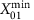=5 μm of the dynamic range of the control space. The upper limit is empirically given by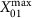=45 μm, because the diffraction efficiency decreases as k1 increases. Note that the entrance pupil diameter of the objective lens is D2NAF3 and the initial beam diameter 2 W=4 mm at the SLM is (de)magnified by the ratio F2/F1=1 to fit with D for optimal performance, which results in X01=D/2NA × k1/k, independent of the focal lengths of the system. In our experiment, a safe working area of 26 × 26 μm2 is used for the optical tweezer patterns and the imaging plane. The discrete phase induces a beam steering error41 but the amount of the error is only 4 nm in our system with 256 grey levels. Thus the resolution is limited by 4 nm, which is much smaller than the long term drift (100 nm) and the LC-SLM refresh fluctuation (100 nm).

The probability for an atom initially trapped in a potential U to escape from an adiabatically lowered potential U′ is approximately given by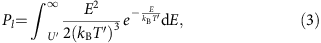where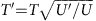is the temperature of the Boltzman distribution26. In our experiment, the lowest trap potential is given by U′=(2R−1)2U. The solid lines in Fig. 5d are the numerically obtained results of equation (3) for T=U/13 and T=U/10, respectively.

### Intensity flicker estimation

The finite modulation depth (0≤ϕ≤Φ) of the SLM phase restricts the ideal linear phase to a modulated phase in a sawtooth shape. We consider two SLM phases ϕ1(x)=mod(k1x+Φ/2, Φ) and ϕ2(x)=mod(k2x+Φ/2, Φ), where Φ2πN, x[−D/2, D/2], D=4.6 mm is the size of the active SLM window, and we assume k1k2 without loss of generality. The phase evolution from ϕ1(x) to ϕ2(x) is then given by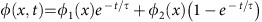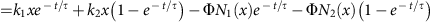, where N1,2(x)=[k1,2x/Φ] is a function defined with the Gauss’ symbol [x]=x−mod(x) and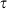denotes the response time. The condition N1(x)=N2(x) defines the flicker-free evolution regions (the shaded regions in Fig. 5a). In our experiment, the flicker-free regions are divided into two regions respectively satisfying N1(x)=N2(x) and N1(x)=N2(x)−1, because R is large enough for an atom transport or (k2k1)(D/2)<2Φ. As a result, R defined by the sum of the flicker-free regions divided by D/2 is given by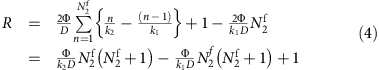for the case of N1(D/2)=N2(D/2)≡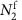and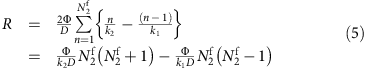for the case of N1(D/2)=N2(D/2). The R in equation (4) can be further simplified to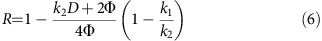under the assumption N1=N2k2D/2/Φ. The obtained analytic result for the R is within 2% difference from the actual numerical value estimated by considering the circular active SLM window, the Gaussian beam profile of the optical tweezers, the 2D nature of the k1 and k2, and the discrete pixel size of the SLM. Within the experimental variation of k1 and k2, R varies from 0.86 to 0.96 as shown in Fig. 5d.

### Single-frame displacement limit

The maximum single-frame displacement ΔX01=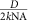Δk is also given as a function of R. When equation (6) is expressed with k2D=2kNAX01 and 1−k1/k2X01/X01, we obtain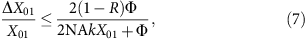so the single-frame displacement is proportional to 1−R.

### The trap loss simulation in Fig. 4b,c

For a pair of initial and final trap potentials, which have N trap sites, two phase holograms are calculated using Gerschberg–Saxton algorithm, respectively. Some of the sites in the initial trap potential are separated by 1/18wo from the final trap potential. The in-between holograms are constructed by ϕ1et/τ+ϕ2(1−et/τ), which generate the transient behaviour of a single trap potential as shown in Fig. 4b. Then, the trajectories (p, q) of the 1D classical Hamiltonian equation of motion calculated by the symplectic Euler method are used to estimate the trap loss probability in Fig. 4c, where we use a loss criteria of |q(t)|>2.5wo and the initial energy and positions are sampled using the Monte-Carlo method.

### Data availability

The data that support the findings of this study are available from the corresponding author upon request.

How to cite this article: Kim, H. et al. In situ single-atom array synthesis using dynamic holographic optical tweezers. Nat. Commun. 7, 13317 doi: 10.1038/ncomms13317 (2016).

Publisher's note: Springer Nature remains neutral with regard to jurisdictional claims in published maps and institutional affiliations.

## References

1. 1

Schlosser, N., Reymond, G., Protsenko, I. & Grangier, P. Sub-poissonian loading of single atoms in a microscopic dipole trap. Nature 411, 1024–1027 (2001).

2. 2

Schlosser, N., Reymond, G. & Grangier, P. Collisional blockade in microscopic optical dipole traps. Phys. Rev. Lett. 89, 023005 (2002).

3. 3

Karski, M. et al. Quantum walk in position space with single optically trapped atoms. Science 325, 174–177 (2009).

4. 4

Preiss, P. M. et al. Strongly correlated quantum walks in optical lattices. Science 347, 1229–1233 (2015).

5. 5

Beugnon, J. et al. Quantum interference between two single photons emitted by independently trapped atoms. Nature 440, 779–782 (2006).

6. 6

Bakr, W. S., Gillen, J. I., Peng, A., Folling, S. & Greiner, M. A quantum gas microscope for detecting single atoms in a Hubbard-regime optical lattice. Nature 462, 74–77 (2009).

7. 7

Miroshnychenko, Y. et al. An atom-sorting machine. Nature 442, 151–151 (2006).

8. 8

Isenhower, L. et al. Demonstration of a neutral atom controlled-NOT quantum gate. Phys. Rev. Lett. 104, 010503 (2006).

9. 9

Kaufman, A. M. et al. Two-particle quantum interference in tunnel-coupled optical tweezers. Science 345, 306–309 (2014).

10. 10

Nelson, K. D., Li, X. & Weiss, D. S. Imaging single atoms in a three-dimensional array. Nat. Phys. 3, 556–560 (2007).

11. 11

Wang, Y., Zhang, X., Corcovilos, T. A., Kumar, A. & Weiss, D. S. Coherent addressing of individual neutral atoms in a 3D optical lattice. Phys. Rev. Lett. 115, 043003 (2015).

12. 12

Nogrette, F. et al. Single-atom trapping in holographic 2D arrays of microtraps with arbitrary geometries. Phys. Rev. X 4, 021034 (2014).

13. 13

Xia, T. et al. Randomized benchmarking of single-qubit gates in a 2D array of neutral-atom qubits. Phys. Rev. Lett. 114, 100503 (2015).

14. 14

Fung, Y., Carpentier, A., Sompet, P. & Andersen, M. Two-atom collisions and the loading of atoms in microtraps. Entropy 16, 582–606 (2014).

15. 15

Lester, B. J., Luick, N., Kaufman, A. M., Reynolds, C. M. & Regal, C. A. Rapid production of uniformly filled arrays of neutral atoms. Phys. Rev. Lett. 115, 073003 (2015).

16. 16

Bakr, W. S. et al. Orbital excitation blockade and algorithmic cooling in quantum gases. Nature 480, 500–503 (2011).

17. 17

Sherson, J. F. et al. Single-atom-resolved fluorescence imaging of an atomic Mott insulator. Nature 467, 68–72 (2010).

18. 18

Weiss, D. S. et al. Another way to approach zero entropy for a finite system of atoms. Phys. Rev. A 70, 040302 (R) (2004).

19. 19

Vala, J. et al. Perfect pattern formation of neutral atoms in an addressable optical lattice. Phys. Rev. A 71, 032324 (2005).

20. 20

Lengwenus, A., Kruse, J., Schlosser, M., Tichelmann, S. & Birkl, G. Coherent transport of atomic quantum states in a scalable shift register. Phys. Rev. Lett. 105, 170502 (2010).

21. 21

Kuhr, S. et al. Coherence properties and quantum state transportation in an optical conveyor belt. Phys. Rev. Lett. 91, 213002 (2003).

22. 22

Kaufman, A. M. et al. Entangling two transportable neutral atoms via local spin exchange. Nature 527, 208–211 (2015).

23. 23

Xu, P. et al. Interaction-induced decay of a heteronuclear two-atom system. Nat. Commun. 6, 7803 (2015).

24. 24

Dorner, U., Calarco, T., Zoller, P., Browaeys, A. & Grangier, P. Quantum logic via optimal control in holographic dipole traps. J. Opt. B Quant. Semiclass. Opt. 7, S341–S346 (2005).

25. 25

Sortais, Y. R. P. et al. Diffraction-limited optics for single-atom manipulation. Phys. Rev. A 75, 013406 (2007).

26. 26

Tuchendler, C., Lance, A. M., Browaeys, A., Sortais, Y. R. P. & Grangier, P. Energy distribution and cooling of a single atom in an optical tweezer. Phys. Rev. A 78, 033425 (2008).

27. 27

Montes-Usategui, M., Pleguezuelos, E., Andilla, J. & Martin-Badosa, E. Fast generation of holographic optical tweezers by random mask encoding of Fourier components. Opt. Express 14, 2101–2107 (2006).

28. 28

Miroshnychenko, Y. et al. Continued imaging of the transport of a single neutral atom. Opt. Express 11, 3498–3502 (2003).

29. 29

Shimobaba, T., Ito, T., Masuda, N., Ichihashi, Y. & Takada, N. Fast calculation of computer-generated-hologram on AMD HD5000 series GPU and OpenCL. Opt. Express 18, 9955–9960 (2010).

30. 30

McGloin, D., Spalding, G. C., Melville, H., Sibbett, W. & Dholakia, K. Applications of spatial light modulators in atom optics. Opt. Express 11, 158–166 (2003).

31. 31

He, X., Xu, P., Wang, J. & Zhan, M. Rotating single atoms in a ring lattice generated by a spatial light modulator. Opt. Express 17, 21007–21014 (2009).

32. 32

Curtis, J. E., Koss, B. A. & Grier, D. G. Dynamic holographic optical tweezers. Opt. Commun. 207, 169–175 (2002).

33. 33

Muldoon, C. et al. Control and manipulation of cold atoms in optical tweezers. New J. Phys. 14, 073051 (2012).

34. 34

Boyer, V. et al. Dynamic manipulation of Bose-Einstein condensates with a spatial light modulator. Phys. Rev. A 73, 031402 (2006).

35. 35

Endres, M. et al. Cold Matter Assembled Atom-by-Atom.. http://arxiv.org/abs/1607.03044 (2016).

36. 36

Barredo, D. et al. An atom-by-atom assembler of defect-free arbitrary 2d atomic arrays. http://arxiv.org/abs/1607.03042 (2016).

37. 37

Lee, W., Kim, H. & Ahn, J. Three-dimensional rearrangement of single atoms using actively controlled optical microtraps. Opt. Express 24, 9816–9825 (2016).

38. 38

Jauregui, R. Nonperturbative and perturbative treatments of parametric heating in atom traps. Phys. Rev. A 64, 053408 (2001).

39. 39

Gehm, M. E., O’Hara, K. M., Savard, T. A. & Thomas, J. E. Dynamics of noise-induced heating in atom traps. Phys. Rev. A 58, 3914–3921 (1998).

40. 40

McGovern, M., Grunzweig, T., Hilliard, A. J. & Andersen, M. F. Single beam atom sorting machine. Laser Phys. Lett. 9, 78–84 (2012).

41. 41

Engstrom, D., Bengtsson, J., Eriksson, E. & Goksor, M. Improved beam steering accuracy of a single beam with a 1D phase-only spatial light modulator. Opt. Express 16, 18275–18287 (2008).

## Acknowledgements

This research was supported by the Samsung Science and Technology Foundation [SSTF-BA1301-12]. The construction of the cold atom apparatus was in part supported by the Basic Science Research Program [2013R1A2A2A05005187] through the National Research Foundation of Korea.

## Author information

Authors

### Contributions

H.K., W.L. and J.A. conceived the research and designed the experiment. H.K., H.-g.L., Y.S. and H.J. constructed the experimental apparatus. H.K. and W.L. performed most of the experiments and the data analysis, with guidance and theoretical support from J.A. All authors contributed to the writing of the manuscript.

### Corresponding author

Correspondence to Jaewook Ahn.

## Ethics declarations

### Competing interests

The authors declare no competing financial interests.

## Rights and permissions

Reprints and Permissions

• ### Preparation of hundreds of microscopic atomic ensembles in optical tweezer arrays

• Yibo Wang
• , Sayali Shevate
• , Tobias Martin Wintermantel
•  & Shannon Whitlock

npj Quantum Information (2020)

• ### Light reflection and transmission in planar lattices of cold atoms

• Sung-Mi Yoo
•  & Juha Javanainen

Optics Express (2020)

• ### Rydberg Atom Entanglements in the Weak Coupling Regime

• Hanlae Jo
• , Yunheung Song
• , Minhyuk Kim
•  & Jaewook Ahn

Physical Review Letters (2020)

• ### Discrete time crystal in a finite chain of Rydberg atoms without disorder

• Chu-hui Fan
• , D. Rossini
• , Han-Xiao Zhang
• , Jin-Hui Wu
• , M. Artoni
•  & G. C. La Rocca

Physical Review A (2020)

• ### Extracting atoms one by one from a small matter-wave soliton

• Fatema Hamodi
•  & Lev Khaykovich

Journal of Physics B: Atomic, Molecular and Optical Physics (2020)